Get inspired by the success stories of our students in IIT JAM MS, ISI  MStat, CMI MSc DS.  Learn More

# Unbiased, Pascal and MLE | ISI MStat 2019 PSB Problem 7This is a problem from the ISI MStat Entrance Examination,2019 involving the MLE of the population size and investigating its unbiasedness.

## The Problem:

Suppose an SRSWOR of size n has been drawn from a population labelled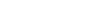, where the population size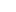is unknown.

(a)Find the maximum likelihood estimator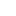of.

(b)Find the probability mass function of.

(c)Show that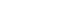is an unbiased estimator of.

## Prerequisites:

(a) Simple random sampling (SRSWR/SRSWOR)

(b)Maximum Likelihood estimator and how to find it.

(c)Unbiasedness of an estimator.

(d)Identities involving Binomial coefficients. (For this, you may refer to any standard text on Combinatorics like R.A.Brualdi,Miklos Bona etc.)

## Solution:

(a) Let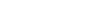be the sample to be selected. In the SRSWOR scheme,

the selection probability of a sample of size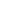is given by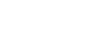.

As,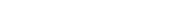, we have the maximum among them , that is theth order statistic,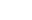is always less than.

Now,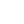is an increasing function of. So, of course,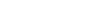, thus on reciprocating, we have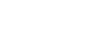. Hence the maximum likelihood estimator ofi.e.is.

(b) We need to find the pmf of.

See that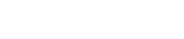, where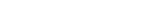.

Can you convince yourself why?

(c) We use a well known identity , the Pascal's Identity to rewrite the distribution of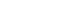a bit more precisely:

We write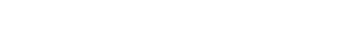Thus, we have :Also, use the Hockey Stick Identity to see that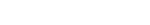So, we have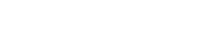.

Thus, we get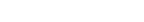## Useful Exercise:

Look up the many proofs of the Hockey Stick Identity. But make sure you at least learn the proof by a combinatorial argument and an alternative proof involving visualizing the identity via the Pascal's Triangle.

This is a problem from the ISI MStat Entrance Examination,2019 involving the MLE of the population size and investigating its unbiasedness.

## The Problem:

Suppose an SRSWOR of size n has been drawn from a population labelled, where the population sizeis unknown.

(a)Find the maximum likelihood estimatorof.

(b)Find the probability mass function of.

(c)Show thatis an unbiased estimator of.

## Prerequisites:

(a) Simple random sampling (SRSWR/SRSWOR)

(b)Maximum Likelihood estimator and how to find it.

(c)Unbiasedness of an estimator.

(d)Identities involving Binomial coefficients. (For this, you may refer to any standard text on Combinatorics like R.A.Brualdi,Miklos Bona etc.)

## Solution:

(a) Letbe the sample to be selected. In the SRSWOR scheme,

the selection probability of a sample of sizeis given by.

As,, we have the maximum among them , that is theth order statistic,is always less than.

Now,is an increasing function of. So, of course,, thus on reciprocating, we have. Hence the maximum likelihood estimator ofi.e.is.

(b) We need to find the pmf of.

See that, where.

Can you convince yourself why?

(c) We use a well known identity , the Pascal's Identity to rewrite the distribution ofa bit more precisely:

We writeThus, we have :Also, use the Hockey Stick Identity to see thatSo, we have.

Thus, we get## Useful Exercise:

Look up the many proofs of the Hockey Stick Identity. But make sure you at least learn the proof by a combinatorial argument and an alternative proof involving visualizing the identity via the Pascal's Triangle.

This site uses Akismet to reduce spam. Learn how your comment data is processed.

### Knowledge Partner